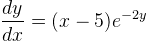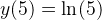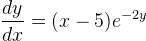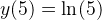# Trouble with particular solution of differential equation - rewrite my answer?

cowmoo32
Trouble with particular solution of differential equation - [SOLVED]

## Homework Statement

Find the particular solution of the differential equationsatisfying the initial condition## The Attempt at a Solution

[STRIKE]I end up with (1/2)ln(-x^2+10x) which does satisfy the initial conditions, yet it's coming back as incorrect. Is there another way I can write the formula?[/STRIKE]

I figured it out, check post #3

Last edited:

Mentor

## Homework Statement

Find the particular solution of the differential equationsatisfying the initial condition## The Attempt at a Solution

I end up with (1/2)ln(-x^2+10x) which does satisfy the initial conditions, yet it's coming back as incorrect. Is there another way I can write the formula?
Show how you got the result you show. I assume you mean y = (1/2)ln(-x2 + 10x).

Satisfying the initial conditions is not enough: the solution has to satisfy the differential equation, too.

cowmoo32
Scratch that above, I had a negative out of place. See below. I corrected it and it's coming back as correct.

$\frac{dy}{dx}$=e-2x(x-5)

$\int$ (e2y)dy = $\int (x-5)dx$

e2y = x2 - 10x + C

2y = ln(x2 - 10x + C)

C = 50

Last edited: